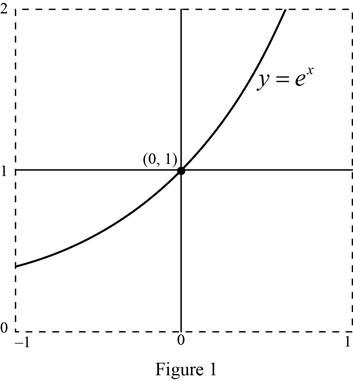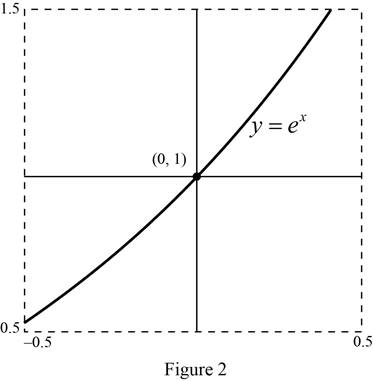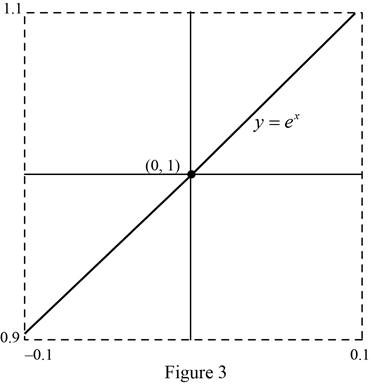# The graph of the curve y = e x in the view of rectangle [ − 1 , 1 ] by [ 0 , 2 ] , [ − 0.5 , 0.5 ] by [ 0.5 , 1.5 ] and [ − 0.1 , 0.1 ] by. [ 0.9 , 1.1 ] .### Single Variable Calculus: Concepts...

4th Edition
James Stewart
Publisher: Cengage Learning
ISBN: 9781337687805### Single Variable Calculus: Concepts...

4th Edition
James Stewart
Publisher: Cengage Learning
ISBN: 9781337687805

#### Solutions

Chapter 2.6, Problem 2E
To determine

## To sketch: The graph of the curve y=ex in the view of rectangle [−1,1] by [0,2], [−0.5,0.5] by [0.5,1.5] and [−0.1,0.1] by.[0.9,1.1].

Expert Solution

### Explanation of Solution

Graph:

Use the online graphing calculator to draw the graph of the function y=ex in the view of rectangle [1,1] by [0,2] as shown below in Figure 1.Graph:

Use the online graphing calculator to draw the graph of the function y=ex in the viewing rectangle [0.5,0.5] by [0.5,1.5] as shown below in Figure 2.Graph:

Use the online graphing calculator to draw the graph of the function y=ex in the viewing rectangle [0.1,0.1] by.[0.9,1.1] as shown below in Figure 3.Observe that the graphs, the curve looks almost like a straight line as viewing rectangle toward the point (0,1). In other words, the curve becomes almost identical from its tangent line as viewing rectangle gets smaller.

### Have a homework question?

Subscribe to bartleby learn! Ask subject matter experts 30 homework questions each month. Plus, you’ll have access to millions of step-by-step textbook answers!